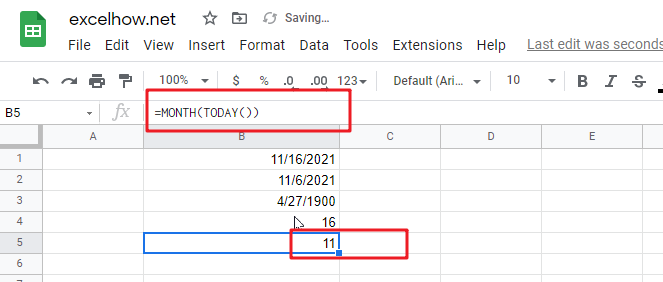# ExcelHow

This post will guide you how to use Google Sheets TODAY function with syntax and examples.

## Description

The Google Sheets TODAY function get the current date as a date value. And it will be updated each time when your worksheet is changed or opened. This function does not need to add any arguments.

You can use the TODAY function to get the current date displayed on your worksheet. And it also can be used for calculating intervals. For example, if you want to know that person’s age (he was born in 1984), you can use the following formula:

=YEAR(TODAY())-1984

This formula will use the TODAY function as an argument for the YEAR function to get the current year, and then subtracts 1984, getting his age.

The TODAY function is a build-in function in Google Sheets and it is categorized as a Date function.

## Syntax

The syntax of the TODAY function is as below:

=TODAY()

There are no any arguments for the TODAY function, but you still need to use empty parentheses ().

Note:

• TODAY function will be updated on every edit made to the spreadsheet and it will impact spreadsheet performance.
• TODAY function will create a date without the current time, and if you wish to get the current date and time, and you can use the NOW function.
• TODAY function will always get the current date and the last date in the spreadsheet was recalculated, rather than remaining at the date when it was first entered.

## Google Sheets TODAY Function Examples

The below examples will show you how to use google sheets TODAY Function to return the current system date.

1# using the TODAY function to get the current date, enter the following formula in Cell B1.

`=TODAY()`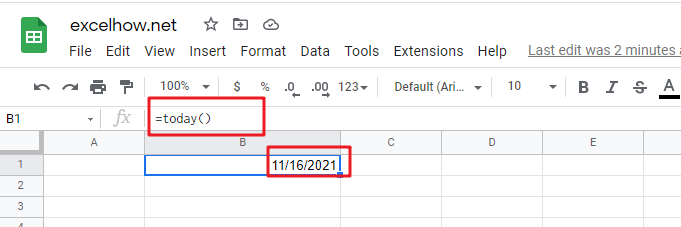2# Get the current date and then subtracts 10 days, using the following formula:

`=TODAY() -10`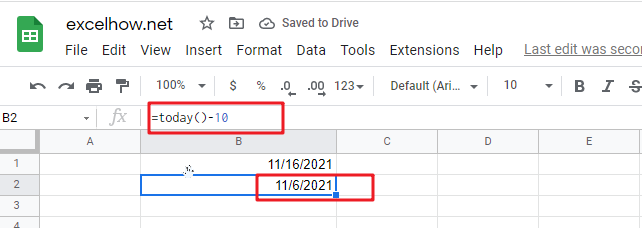3# Get the number of days between the current date and 03/14/2022, type the following formula:

`=DATEVALUE("03/14/2019")-TODAY()`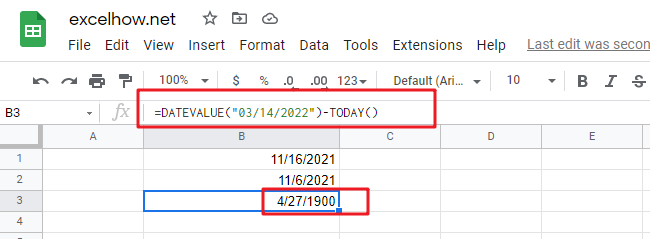4# Get the current day of the month, using the following formula:

`=DAY(TODAY())`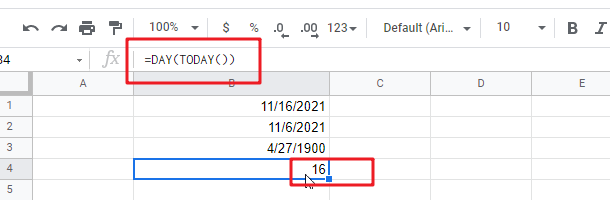5# Get the current month of the year, type the following formula in Cell B1:

`=MONTH(TODAY())`